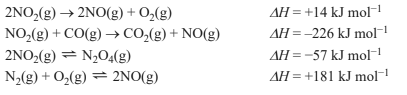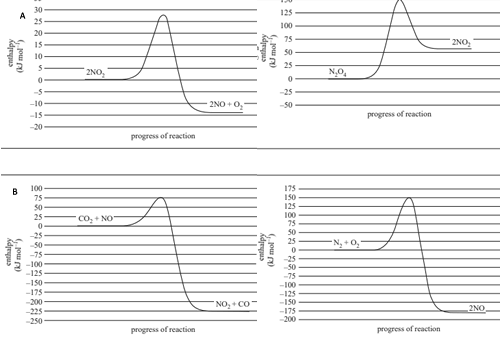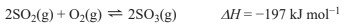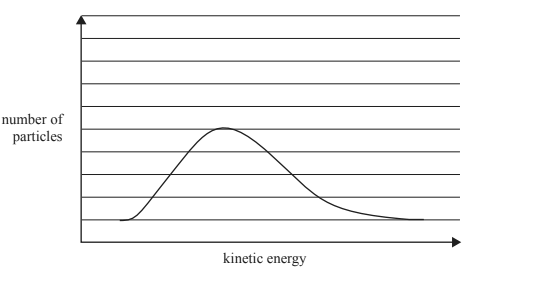Enthalpy (2021 VCE ) 1) Butane, C4H10, undergoes complete combustion according to the following equation. 2C4H10(g) + 13O2(g) → 8CO2(g) + 10H2O(g) 67.0 g of C4H10 released 3330 kJ of energy during complete combustion at standard laboratory conditions (SLC). The mass of carbon dioxide, CO2, produced was A. 0.105 g B. 3.18 g C. 50.9 g D. 204 g Solution2) Consider the following chemical equations.Which one of the following graphs is consistent with the chemical equations above?Solution3)Which one of the following statements describes the effect that adding a catalyst will have on the energy profile diagram for an exothermic reaction? A. The energy of the products will remain the same. B. The shape of the energy profile diagram will remain the same. C. The peak of the energy profile will move to the left as the reaction rate increases. D. The activation energy will be lowered by the same proportion in the forward and reverse reactions.   Solution4) The reaction for the oxidation of sulfur dioxide, SO2 , is shown below.a. 1.00 mol of SO2 and 1.00 mol of oxygen, O2 , are placed into an evacuated, sealed 3.00 L container at 100 °C. After the reaction reaches equilibrium, the container contains 20.0 g of sulfur trioxide, SO3 . Calculate the equilibrium constant, Kc, for this reaction at 100 °C.     Solutionb. The graph below shows the Maxwell-Boltzmann distribution curve for the SO3 molecules in the container at a particular temperature. On the graph, draw the Maxwell-Boltzmann distribution curve of SO3 at a significantly lower temperature.Solution c. The volume of the closed container is doubled. Describe the effect that this has on the concentration of SO2 from the time just before the volume was changed until after the system re-established its equilibrium. Solution